# Getting Started with ASP.NET Core Chart

8 Jun 20218 minutes to read

This section explains the steps required to populate the chart with data, data labels, tooltips, and title. This section covers only minimal features that needed to get started with the Chart.

In this tutorial, you can learn how to create a simple chart. The following screenshot displays the output after completing this tutorial.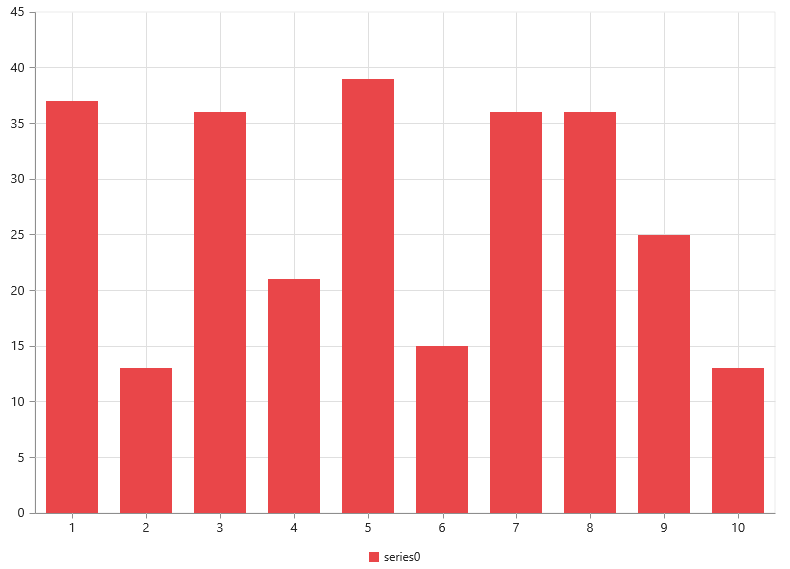Simple chart

1. Create a new ASP.NET core project. Please refer to ASP.Net core 1.0-Getting Started documentation to create new project, and add necessary DLL’s and script files.

2. Add the following code in index.cshtml file to create the Chart control in View page.

• CSHTML
• ``````<div>

<ej-chart id="chart"></ej-chart>

</div>``````

Now, the Chart is rendered with auto-generated random values and default column chart type.

Initialize the chart by using the ej-chart tag. The chart is rendered to the size of its container, by default. You can also customize the chart dimension either by setting the width and height of the container element as in the above code example, or by using the Size option of the chart.

## Populate chart with data

Now, you can see how to plot data source to the chart. First, generate a data source with following fields in controller – Month and Sales.

• CSHARP
• ``````public class HomeController : Controller
{
public IActionResult Index()
{
//// Create dataSource to chart
List<ChartData> data = new List<ChartData>();
///...
ViewBag.ChartData = data;
return View();
}
}

public class ChartData
{
public string Month;
public double Sales;
public ChartData(string month, double sales)
{
this.Month = month;
this.Sales = sales;
}
}``````

Add a series to the chart by using Series option, and set the chart type as Line using type option.

• CSHTML
• ``````<ej-chart id="container">
<e-chart-series>
<e-series type="Line"></e-series>
</e-chart-series>
</ej-chart>``````

Next, map the month and sales values in the data source to the line series by setting XName and YName with the field names respectively, and then set the actual data by using DataSource option.

• CSHTML
• ``````<ej-chart id="container">
<e-chart-series>
<e-series datasource="ViewBag.ChartData" x-name="Month" y-name="Sales" type="Line"></e-series>
</e-chart-series>
<e-size height="400" width="600"></e-size>
</ej-chart>``````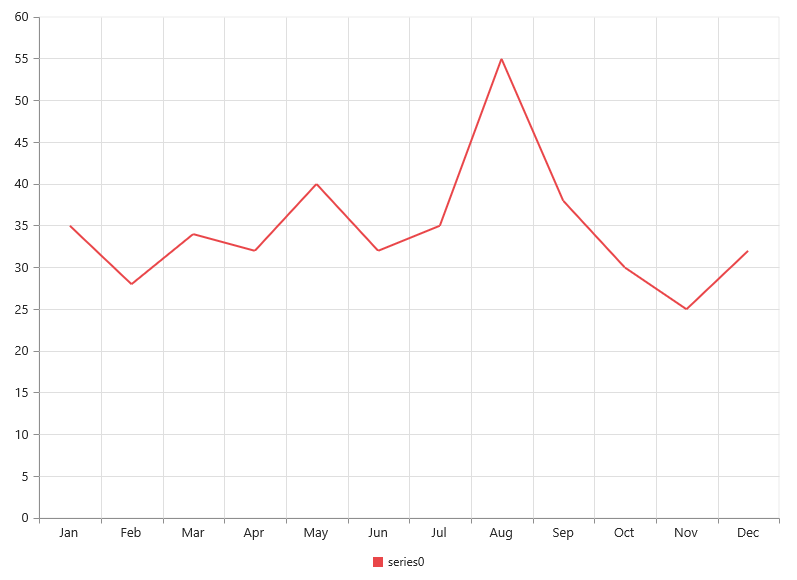Since the data is related to sales, format the vertical axis labels by adding ‘\$’ as prefix and ‘K’ as suffix to each label. This can be achieved by setting the “\${value}K” to the label-format option of the axis. Here, {value} acts as a placeholder for each axis label.

The following code example illustrates this,

• CSHTML
• ``````<ej-chart id="container">

<e-primary-y-axis label-format="\${value}k">
</e-primary-y-axis>

</ej-chart>``````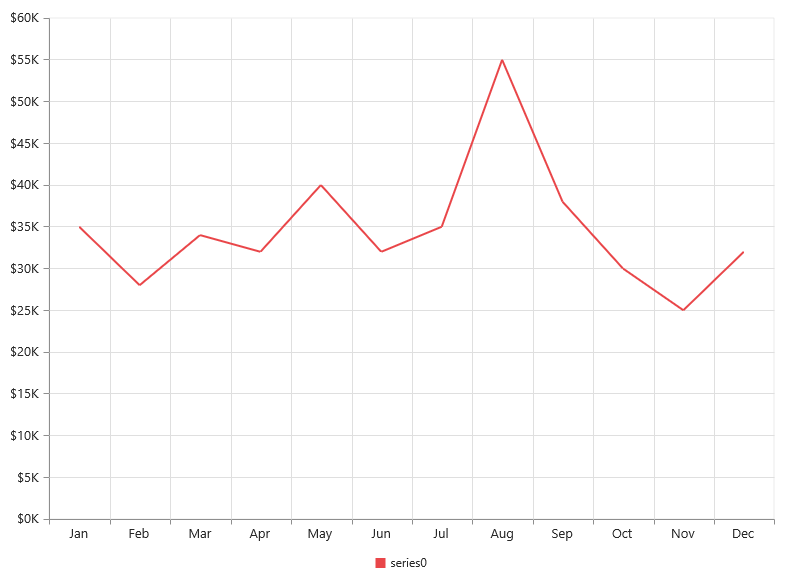You can add data labels to improve the readability of the chart. This can be achieved by enabling the Visible option in the data-label option. Now, the data labels are rendered at the top of all the data points.

The following code example illustrates this,

• CSHTML
• ``````<ej-chart id="container">
<e-chart-series>
<e-series>
<e-marker>
<e-data-label visible="true">
</e-data-label>
</e-marker>
</e-series>
</e-chart-series>
</ej-chart>``````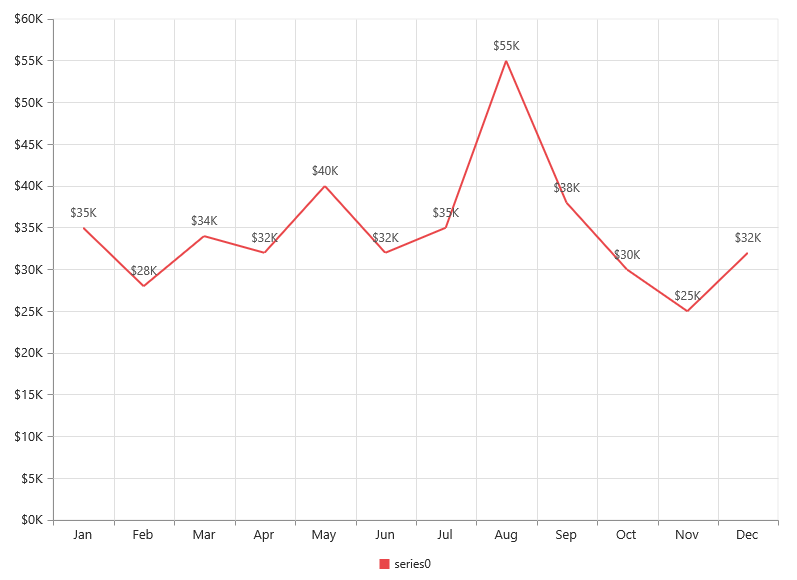There are situations where the default label content is not sufficient to the user. In this case, use the template option to format the label content with some additional information.

• HTML
• ``````<!DOCTYPE html>
<html>
<body>
<div id="point">#point.x#:\$#point.y#K</div>
</div>
</body>
</html>``````

The above HTML template is used as a template for each data label. Here, “point.x” and “point.y” are the placeholder text that are used to display the corresponding data point’s x & y values.

The following code example illustrates how to set the ID of the above template to data-label template option,

• CSHTML
• ``````<ej-chart id="container">
<e-chart-series>
<e-series>
<e-marker>
<e-data-label visible="true" template="dataLabelTemplate">
</e-data-label>
</e-marker>
</e-series>
</e-chart-series>
</ej-chart>``````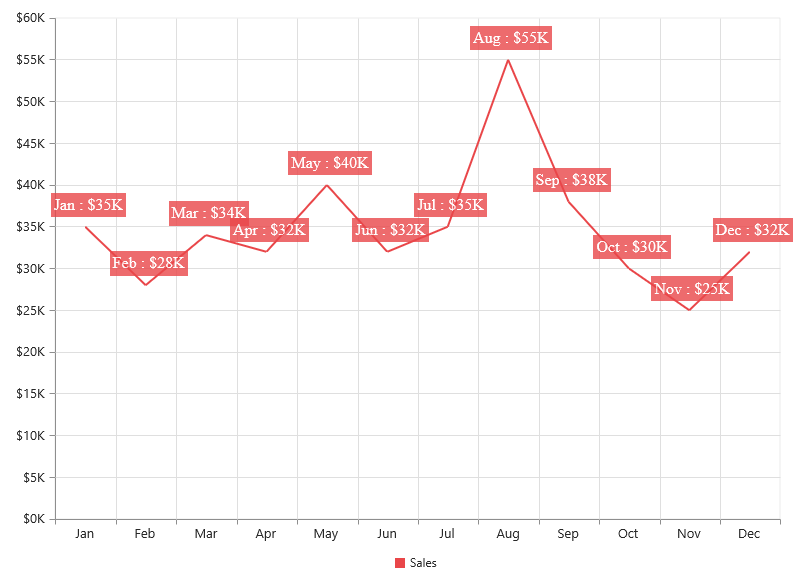## Enable legend

You can enable or disable the legend by using the Visible option in the legend option. It is enabled in the chart, by default.

• CSHTML
• ``````<ej-chart id="container">
<e-chart-series>
<e-series name="Sales">
</e-series>
</e-chart-series>
<e-legend visible="true"></e-legend>
</ej-chart>``````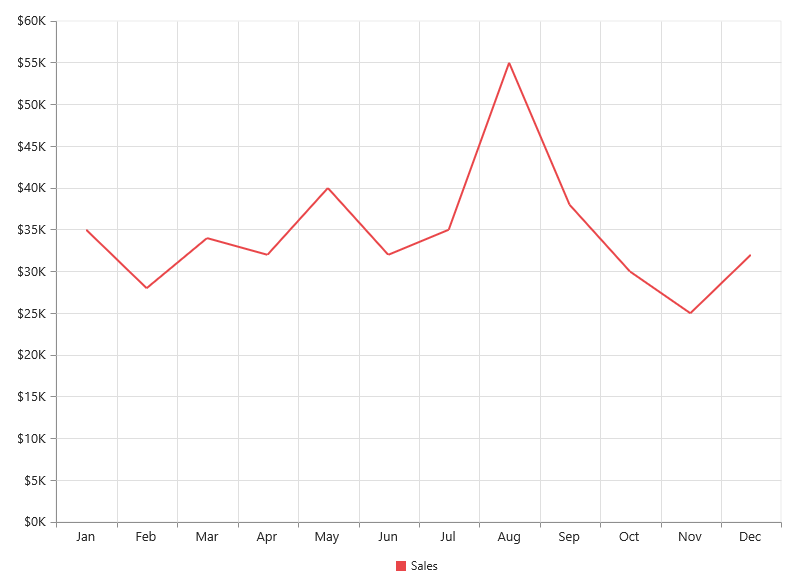## Enable tooltip

The Tooltip is used when the information cannot be displayed by using the data-label option due to space constraints. You can enable the tooltip by using the Visible option of the chart-tooltip option in the specific series.

The following code example illustrates this,

• CSHTML
• ``````<ej-chart id="container">
<e-chart-series>
<e-series>
<e-chart-tooltip visible="true"></e-chart-tooltip>
</e-series>
</e-chart-series>
</ej-chart>``````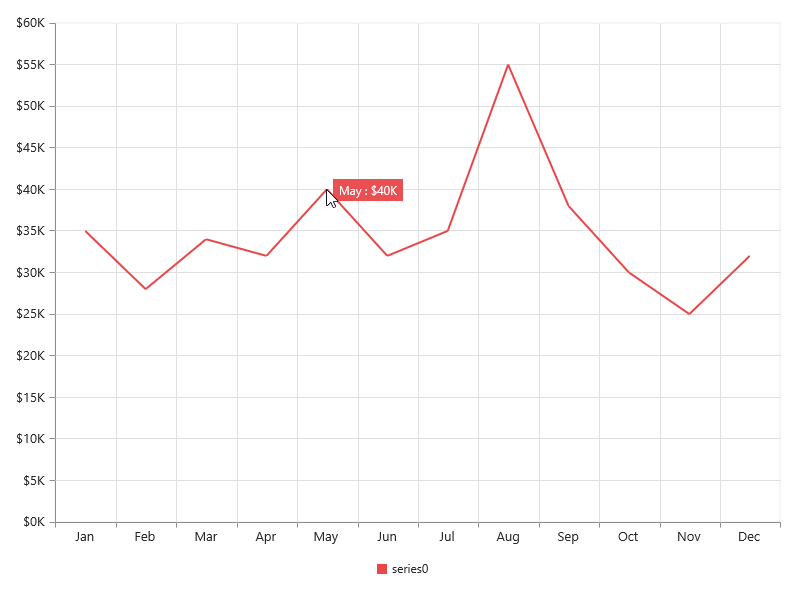• ``````<ej-chart id="container">